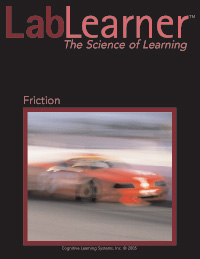# FrictionFriction

Through investigation and the use of mathematical formulas, students begin to define the relationship between speed, velocity, and acceleration. Students begin to understand that velocity is a measure of speed and direction, and students find that an object with no change in velocity has no acceleration.

Investigations that involve pulling a wood block along a table at a constant velocity are performed. Using the formula F = ma, students discover that the sum of the forces on an object with constant velocity is zero. Using this knowledge, students determine the frictional force on a wood block and a wood box in a variety of scenarios.

Through investigations, students discover that frictional force and applied forces affect the motion of an object. Further investigating frictional force, they find that it is dependent on the weight of the object being moved and on the smoothness of the surfaces in contact with one another. Also, students find that frictional force is independent of the surface area of the object being moved and the velocity at which the object moves. Through these investigations, students begin to draw conclusions about the way forces affect motion.

Investigation focus:

• Observe the effects of frictional force on motion.
• An object with constant velocity has zero acceleration.
• An object with increased velocity while moving away from the origin has positive acceleration.
• An object with decreased velocity while moving away from the origin has negative acceleration.
• Realize that friction is a force.
• Find that when an object is moved with zero acceleration, frictional force is equal and opposite to the applied force.
• Use the formula F = ma to calculate the sum of the forces.
• Apply the Law of Conservation of Matter and Energy to the movement of a wood block.
• Explore velocity and understand that velocity is a measure of speed in a given direction.
• Through investigation, students will discover that velocity does not affect the amount of frictional force between two objects.
• Calculate the weight of an object using the formula weight = mass ◊ acceleration due to gravity.
• Find that the frictional force between an object and a surface depends on the weight of the object.
• Discover that the frictional force between an object and a surface is independent of the surface area of the object.
• Find that the frictional force between an object and a surface depends on the smoothness of the combination of surfaces.